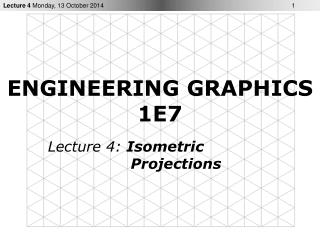DownloadDownload PresentationENGINEERING GRAPHICS 1E7

# ENGINEERING GRAPHICS 1E7

Download Presentation## ENGINEERING GRAPHICS 1E7

- - - - - - - - - - - - - - - - - - - - - - - - - - - E N D - - - - - - - - - - - - - - - - - - - - - - - - - - -
##### Presentation Transcript

1. ENGINEERING GRAPHICS1E7 Lecture 4: Isometric Projections

2. Irregular Objects • Make an Isometric Drawing of the following irregular object (pyramid)

3. Irregular Objects • OA and OB offsets help to locate apex O • Complete box construction may not be needed in each case

4. Objects with Circular Geometry A circle in a orthographic projection will appear as an ellipse in an isometric drawing. Instead of actual ellipses often approximate ellipses are drawn for isometric drawing. Four-centre ellipses are used to approximate ellipses on isometric planes. How to draw four-centre ellipse???

5. Approximate Ellipse Draw  the  isometric centre  lines  of  the circle. Using  the  centre  lines,  draw an isometric square with sides equal to the diameter of  the  circle. From  the  near  corners  of  the  box,  draw two large arcs with radius R, using the two red points as centres. Draw the two smaller arcs with radius r, using two green points as centres.

6. Cylinder

7. Objects with Circular Geometry

8. Objects with Non-Circular Curved Surfaces • Make an Isometric Drawing of the following curved object

9. Objects with Non-Circular Curved Surfaces • A line that appears as a noncircular curve in a normal orthographic view of an object appears as a non-isometricline in an isometric drawing. • Curves may be drawn using a series of points by measuring along the normal lines in the  orthographic  view  (offset measurements) and  transferring  these points on isometric drawing. Accuracy increases with number of points.

10. Notes • Axonometric projection shows all 3 dimensions, length, width and height • The isometric lines are only drawn to scale. • Inclined and oblique surfaces are drawn using end coordinates • Angles, irregular curves require special techniques • Box construction and offset measurements are common methods